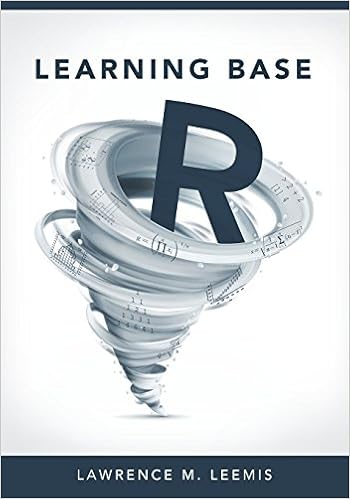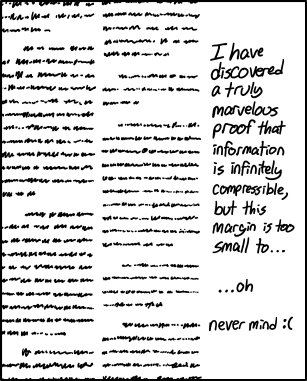## operation precisely impossible

Posted in Books, Kids, R, University life with tags , , , , , , , , , , , , on May 13, 2023 by xi'anSince the solution to the previous riddle from The Riddler on the maximum of  different terms in the composed operation

a∅b∅c∅d∅e∅f

depending on the bracketing ordering and the meaning of each ∅ among one of the six elementary operations got posted today as 974,860, I got back to my R code to understand why it differed from my figures by two orders of magnitude and realised I was overly trusting the R function unique. As it was returning more “different” entries than it should have, especially when choosing the six starting numbers (a,…,f) as Uniform (0,1). Using integers instead led for instance to 946,558, which was not so far from the target. But still imprecise as to whether or not some entries had been counted several times. I mentioned the issue to Robin, who rose to the challenge and within minutes came up with using the R function almost.unique from the CRAN package bazar, then producing outcomes like 974,513, hence quite close to 974,860 for random initialisations!

## learning base R [book review]

Posted in Books, Kids, Statistics, University life with tags , , , , , , , , , , , , , , , , on February 26, 2022 by xi'anThis second edition of an introductory R book was sent to me by the author for a potential CHANCE book review.  As there are many (many) books in the same spirit, the main question behind my reading it (in one go) was on the novelty it brings. The topics Learning Base R covers are

• arithmetics with R
• data structures
• built-in and user-written R functions
• R utilities
• more data structures
• comparison and coercion
• lists and data frames
• resident R datasets
• R interface
• probability calculations in R
• R graphics
• R programming
• simulations
• statistical inference in R
• linear algebra
• use of R packages

within as many short chapters. The style is rather standard, that is, short paragraphs with mostly raw reproductions of line commands and their outcome. Sometimes a whole page long of code examples (if with comments). All in all I feel there are rather too few tables when compared with examples, at least for my own taste. The exercises are mostly short and, while they vary in depth, they show that the book is rather intended for students with some mathematical background (e.g., with a chapter on complex numbers and another one on linear algebra that do not seem immediately relevant for most intended readers). Or more than that, when considering one (of several) exercise (19.30) on the Black-Scholes process that mentions Brownian motion. Possibly less appealing for would-be statisticians.

I also wonder at the pedagogical choice of not including and involving more clearly graphical interfaces like R studio as students are usually not big fans of “old-style” [their wording not mine!] line command languages. For instance, the chapter on packages would have benefited from this perspective. Nothing on Rmarkdown either. Apparently nothing on handling big data, more advanced database manipulation, the related realistic dangers of memory freeze and compulsory reboot, the intricacies of managing different directories and earlier sessions, little on the urgency of avoiding loops (p.233) by vectorial programming, a paradoxically if function being introduced after ifelse, and again not that much on statistics (with density only occurring in exercises).The chapter on customising R graphics may possibly scare the intended reader when considering the all-in-one example of p.193! As we advance though the book, the more advanced examples often are fairly standard programming ones (found in other language manuals) like creating Fibonacci numbers, implementing Eratosthenes sieve, playing the Hanoi Tower game… (At least they remind me of examples read in the language manuals I read as a student.) The simulation chapter could have gone into the one (Chap. 19) on probability calculations, rather than superfluously redefining standard distributions. (Except when defining a random number as a uniformly random number (p.162).)  This chapter also spends an unusual amount of space on linear congruencial pseudo-random generators, while missing to point out the trivia that the randu dataset mentioned twice earlier is actually an outcome from the infamous RANDU Fortran generator. The following section in that chapter is written in such a way that it may give the wrong impression that one can find the analytic solution from repeated Monte Carlo experiments and hence the error. Which is rarely the case, even in finite environments with rational expectations, as one usually does not know of which unit fraction the expectation should be a multiple of. (Remember the Squid Games paradox!) And no mention is made of the prescription of always returning an error estimate along with the numerical approximation. The statistics chapter is obviously more developed, with descriptive statistics, ecdf, but no bootrstap, a t.test curiously applied to the Michelson measurements of the speed of light (how could it be zero?!), ANOVA, regression handled via lm and glm, time series analysis by ARIMA models, which I hope will not be the sole exposure of readers to these concepts.

In conclusion, there is nothing critically wrong with this manual introducing R to newcomers and I would not mind having my undergraduate students reading it (rather than our shorter and home-made handout, polished along the years) before my first mathematical statistics lab. However I do not find it massively innovative in its presentation or choice of concept, even though the most advanced examples are not necessarily standard, and may not appeal to all categories of students.

[Disclaimer about potential self-plagiarism: this post or an edited version will eventually appear in my Book Review section in CHANCE.]

## likelihood inference with no MLE

Posted in Books, R, Statistics with tags , , , , on July 29, 2021 by xi'an

“In a regular full discrete exponential family, the MLE for the canonical parameter does not exist when the observed value of the canonical statistic lies on the boundary of its convex support.”

Daniel Eck and Charlie Geyer just published an interesting and intriguing paper on running efficient inference for discrete exponential families when the MLE does not exist.  As for instance in the case of a complete separation between 0’s and 1’s in a logistic regression model. Or more generally, when the estimated Fisher information matrix is singular. Not mentioning the Bayesian version, which remains a form of likelihood inference. The construction is based on a MLE that exists on an extended model, a notion which I had not heard previously. This model is defined as a limit of likelihood values$\lim_{n\to\infty} \ell(\theta_n|x) = \sup_\theta \ell(\theta|x) := h(x)$

called the MLE distribution. Which remains a mystery to me, to some extent. Especially when this distribution is completely degenerate. Examples provided within the paper alas do not help, as they mostly serve as illustration for the associated rcdd R package. Intriguing, indeed!

## Fermat’s Riddle

Posted in Books, Kids, R with tags , , , , , , , , , , on October 16, 2020 by xi'an

·A Fermat-like riddle from the Riddler (with enough room to code on the margin)

An  arbitrary positive integer N is to be written as a difference of two distinct positive integers. What are the impossible cases and else can you provide a list of all distinct representations?

Since the problem amounts to finding a>b>0 such that$N=a^2-b^2=(a-b)(a+b)$

both (a+b) and (a-b) are products of some of the prime factors in the decomposition of N and both terms must have the same parity for the average a to be an integer. This eliminates decompositions with a single prime factor 2 (and N=1). For other cases, the following R code (which I could not deposit on tio.run because of the packages R.utils!) returns a list

library(R.utils)
library(numbers)
bitz<-function(i,m) #int2bits
c(rev(as.binary(i)),rep(0,m))[1:m]
ridl=function(n){
a=primeFactors(n)
if((n==1)|(sum(a==2)==1)){
print("impossible")}else{
m=length(a);g=NULL
for(i in 1:2^m){
b=bitz(i,m)
if(((d<-prod(a[!!b]))%%2==(e<-prod(a[!b]))%%2)&(d<e))
g=rbind(g,c(k<-(e+d)/2,l<-(e-d)/2))}
return(g[!duplicated(g[,1]-g[,2]),])}}


For instance,

> ridl(1456)
[,1] [,2]
[1,]  365  363
[2,]  184  180
[3,]   95   87
[4,]   59   45
[5,]   40   12
[6,]   41   15


Checking for the most prolific N, up to 10⁶, I found that N=6720=2⁶·3·5·7 produces 20 different decompositions. And that N=887,040=2⁸·3²·5·7·11 leads to 84 distinct differences of squares.

## the limits of R

Posted in Books, pictures, R, Statistics with tags , , , , , , , , , , , , on August 10, 2020 by xi'anIt has been repeated many times on many platforms, the R (or R⁰) number is not a great summary about the COVID-19 pandemic, see eg Rossman’s warning in The Conversation, but Nature chose to stress it one more time (in its 16 Jul edition). Or twice when considering a similar piece in Nature Physics. As Boris Johnson made it a central tool of his governmental communication policy. And some mayors started asking for their own local R numbers! It is obviously tempting to turn the messy and complex reality of this planetary crisis into a single number and even a single indicator R<1, but it is unhelpful and worse, from the epidemiology models being wrong (or at least oversimplifying) to the data being wrong (i.e., incomplete, biased and late), to the predictions being wrong (except for predicting the past). Nothing outrageous from the said Nature article, pointing out diverse degrees of uncertainty and variability and stressing the need to immediately address clusters rather than using the dummy R. As an aside, the repeated use of nowcasting instead of forecasting sounds like a perfect journalist fad, given that it does not seem to be based on a different model of infection or on a different statistical technique. (There is a nowcasting package in R, though!) And a wee bit later I have been pointed out at an extended discussion of an R estimation paper on Radford Neal’s blog.